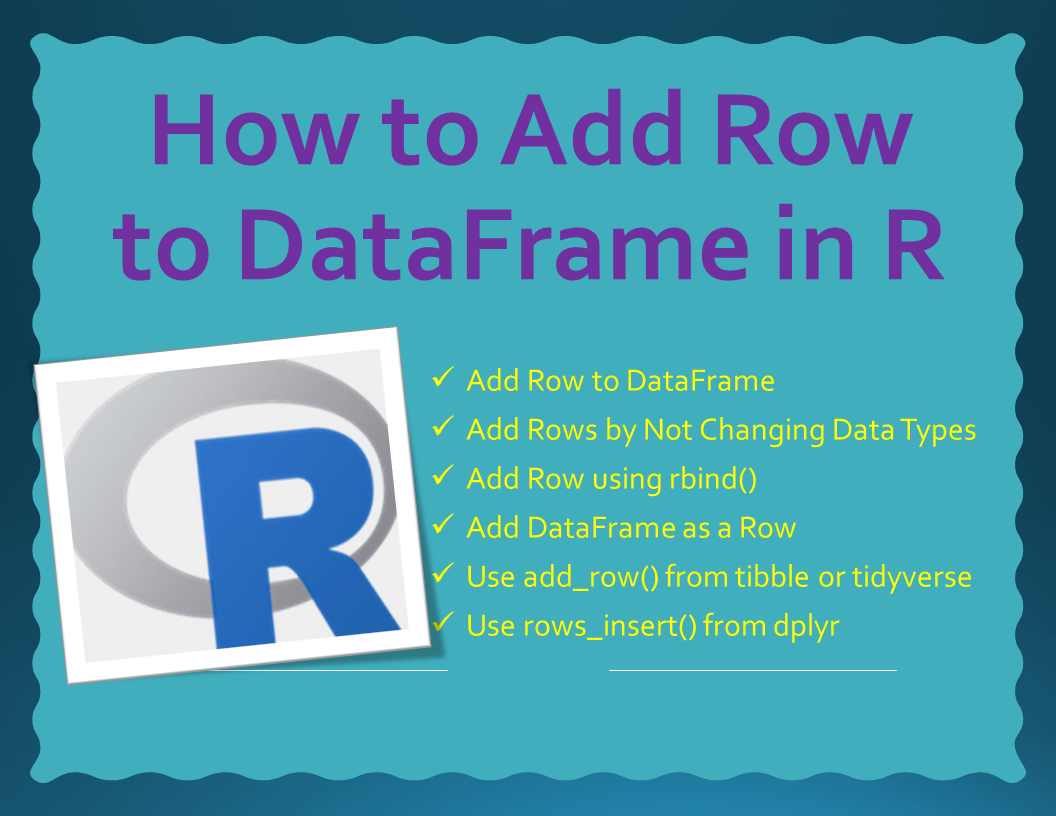# How to Add Row to DataFrame in R?

In order to add a new row to DataFrame in R, first, you need to create either a vector or a list with the row values. And assign this to the DataFrame to add a row. In this article, I will explain how easy to add rows to the DataFrame.

The following methods are used to add a row to DataFrame in R.

## 1. Quick Examples of Add Row to DataFrame

The following are quick examples of how to add rows to a data.frame in R

``````
# Quick Examples

# Add Row to the DataFrame
# This converts all types to string
df[nrow(df) + 1,] <- c(33, 50, "java")

# Add Row to the DataFrame
# with out changing data types
df[nrow(df) + 1,] <- list(33, 50, "java")

new_row = c(id = 33, pages=50, name = "java")
df3 = rbind(df,new_row)

df2 = data.frame(id = 33, pages=50, name = "java")
df3 = rbind(df,df2)

# using tibble/tidyverse
library(tidyverse)
df2 <- df %>%
add_row(id = 33, pages=50, name = "java")

# Using dplyr
library(dplyr)
df2 = data.frame(id = 33, pages=50, name = "java")
df3 <- df %>%
rows_insert(df2)
``````

Let’s create an R DataFrame, run these examples and explore the output. If you already have data in CSV you can easily import CSV files to R DataFrame. Also, refer to Import Excel File into R.

``````
# Create dataframe
df = data.frame(id=c(11,22),
pages=c(32,45),
name=c("spark","python"))
df

# Output
#  id pages   name
#1 11    32  spark
#2 22    45 python
``````

## 2. Add Row to DataFrame

To add a new row to the DataFrame (data.frame) in R, first, you need to get the number of rows in the existing data frame using `nrows(df)` and add this new row towards the end `nrow(df) + 1`. The following example adds a new row at the end of the dataframe.

``````
# Add Row to the DataFrame
# This converts all types to string
df[nrow(df) + 1,] <- c(33, 50, "java")
df

# Output
#  id pages   name
#1 11    32  spark
#2 22    45 python
#3 33    50   java
``````

When you have mixed data types some columns are numeric and some columns are text, then it converts all columns to text. You can check this by running `str(df)`.

## 3. Add Rows by Not Changing Data Types

Use `list()` instead of a vector to add a row. Adding a list() as a row to the dataframe doesn’t change the types. You can run str(df) and validate the result yourself.

``````
# Add Row to the DataFrame
# with out changing data types
df[nrow(df) + 1,] <- list(33, 50, "java")
df
``````

## 4. Add Row using rbind()

The `rbind()` function in R, short for row-bind, is used to combine vectors, lists, matrices, and data frames by rows. The following example adds a list as a row to the DataFrame.

``````
new_row = list(id = 33, pages=50, name = "java")
df3 = rbind(df,new_row)
df3
``````

## 5. Add DataFrame as a Row

You can also use `rbind()` to append the dataframe. When you do this, you have to set consistent column names for all the data frames you want to append.

``````
df2 = data.frame(id = 33, pages=50, name = "java")
df3 = rbind(df,df2)
df3
``````

## 6. Use add_row() from tibble or tidyverse

The packages tibble or tidyverse provides a function `add_row()` to add a row to DataFrame in R. This is a convenient way to add one or more rows of data to an existing data frame.

In order to use this function first, you need to install R package by using `install.packages("tidyverse")` and load it using the `library("tidyverse")`.

``````
# syntax
add_row(.data, ..., .before = NULL, .after = NULL)
``````

Example

``````
# using add_row() From tibble or tidyverse
library(tidyverse)
df2 <- df %>%
add_row(id = 33, pages=50, name = "java")
df2
``````

## 7. Use rows_insert() from dplyr

Finally, use `rows_insert()` function from the dplyr package to add a row to R DataFrame. You need to install and load this package in order to use this function.

``````
# Using rows_insert() from dplyr
library(dplyr)
df2 = data.frame(id = 33, pages=50, name = "java")
df3 <- df %>%
rows_insert(df2)
df3
``````

## 8. Conclusion

In this article, you have learned how to add a row to DataFraem in R by using rbin() base library, add_row() from tibble or tidyverse, and rows_inser() from dplyr.

## References

### Naveen (NNK)

Naveen (NNK) is a Data Engineer with 20+ years of experience in transforming data into actionable insights. Over the years, He has honed his expertise in designing, implementing, and maintaining data pipelines with frameworks like Apache Spark, PySpark, Pandas, R, Hive and Machine Learning. Naveen journey in the field of data engineering has been a continuous learning, innovation, and a strong commitment to data integrity. In this blog, he shares his experiences with the data as he come across. Follow Naveen @ @ LinkedIn